How Cheenta works to ensure student success?
Explore the Back-Story

# Test of Mathematics Solution Subjective 71 - Real solutionsThis is a Test of Mathematics Solution Subjective 71 (from ISI Entrance). The book, Test of Mathematics at 10+2 Level is Published by East West Press. This problem book is indispensable for the preparation of I.S.I. B.Stat and B.Math Entrance.

Also visit: I.S.I. & C.M.I. Entrance Course of Cheenta

## Problem

Consider the following simultaneous equations in x and y :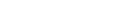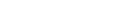where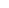is a real constant. Show that these equations admit real solutions in x and y.

## Solution...  (i)... (ii)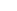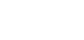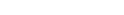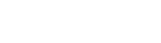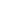[ replacing x in terms of y. ]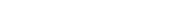This is a 3rd degree polynomial over y.
Now as we know any odd degree polynomial has at least one real root.That is why y has also at least one real root.Now for that particular root we getalso real.
Conclusion:&admits real solutions in x and y.This is a Test of Mathematics Solution Subjective 71 (from ISI Entrance). The book, Test of Mathematics at 10+2 Level is Published by East West Press. This problem book is indispensable for the preparation of I.S.I. B.Stat and B.Math Entrance.

Also visit: I.S.I. & C.M.I. Entrance Course of Cheenta

## Problem

Consider the following simultaneous equations in x and y :whereis a real constant. Show that these equations admit real solutions in x and y.

## Solution...  (i)... (ii)[ replacing x in terms of y. ]This is a 3rd degree polynomial over y.
Now as we know any odd degree polynomial has at least one real root.That is why y has also at least one real root.Now for that particular root we getalso real.
Conclusion:&admits real solutions in x and y.

### Knowledge Partner